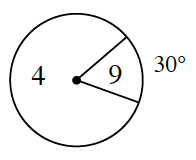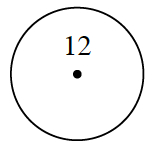### Home > INT2 > Chapter 4 > Lesson 4.1.2 > Problem4-21

4-21.

For each spinner below, calculate the expected value.

1.What is the ratio or likelihood of landing in the $30^\circ$ angle and getting a $9$?
What is the probability of getting a $4$?

Refer to the Math Notes box in Lesson 3.2.1.

1.